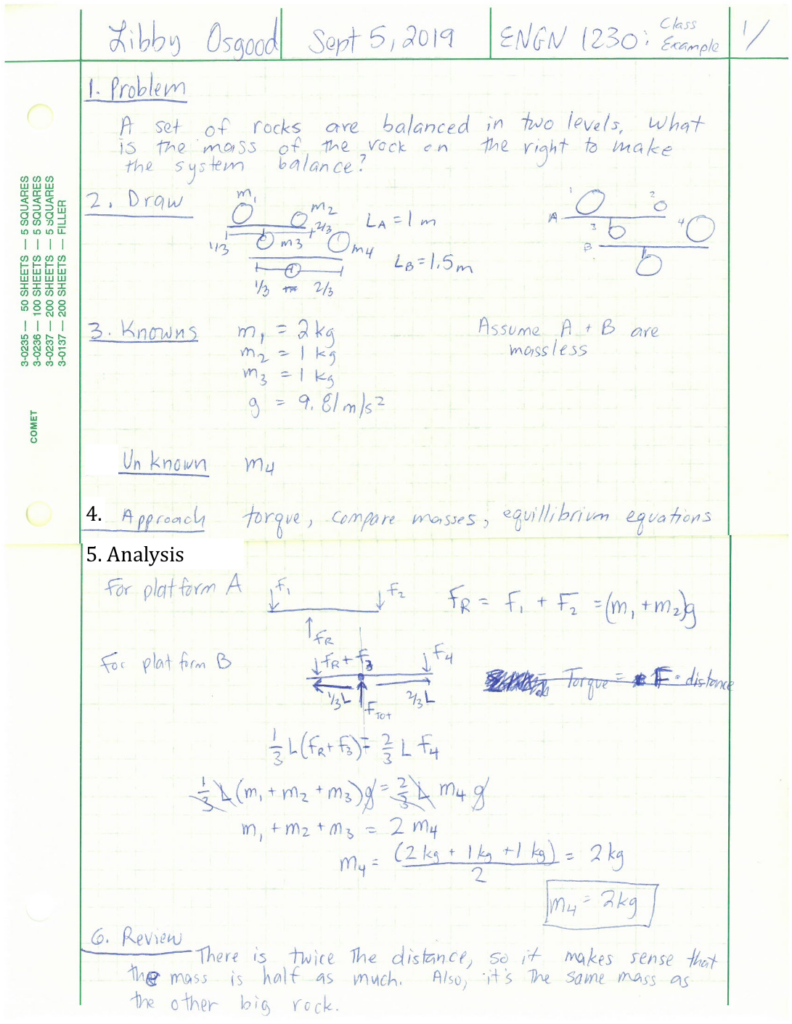Chapter 1: Fundamental Concepts

# 1.7 Problem Solving Process

Learning how to use a structured problem solving process will help you to be more organized and support your future courses. Also, it will train your brain how to approach problems. Just like basketball players practice jump shots over and over to train their body how to act in high pressure scenarios, if you are comfortable and familiar with a structured problem solving process, when you’re in a high pressure situation like a test, you can just jump into the problem like muscle memory.

## 6 Step Problem Solving Method:

1. Problem
• Write out the answer with all necessary information that is given to you. It feels like it takes forever, but it’s important to have the problem and solution next to each other.
2. Draw
• Draw the problem, this is usually a free-body diagram (don’t forget a coordinate frame). Eventually, as you get further into the course, you might need a few drawings. One would be a quick sketch of the problem in the real world, then modelling it into a simplified engineering drawing, and finally the free-body diagram.
3. Known and Unknowns
• Write out a list of the known/given values with the variable and unit, i.e m = 14 kg  (variable = number unit)
• Write out a list of the unknown values that you will have to solve for in order to solve the problem
• You can also add any assumptions you made here that change the problem.
• Also state any constants, i.e. g = 32.2 ft/m2  or g = 9.81 m/s2
• This step helps you to have all of the information in one place when you solve the problem. It’s also important because each number should include units, so you can see if the units match or if you need to convert some numbers so they are all in English or SI. This also gives you the variables side by side to ensure they are unique (so you don’t accidentally have 2 ‘d’ variables and can rename one with a subscript).
4. Approach
• Write a simple sentence or phrase explaining what method/approach you will be using to solve the problem.
• For example: ‘use method of joints’, or equilibrium equations for a rigid body, MMOI for a certain shape, etc.
• This is going to be more important when you get to the later chapters and especially next semester in Dynamics where you can solve the same problem many ways. Might as well practice now!
5. Analysis
• This is the actual solving step. This is where you show all the work you have done to solve the problem.
• When you get an answer, restate the variable you are solving for, include the unit, and put a box around the answer.
6. Review
• Write a simple sentence explaining why (or why not) your answer makes sense. Use logic and common sense for this step.
• When possible, use a second quick numerical analysis to verify your answer. This is the “gut check” to do a quick calculation to ensure your answer is reasonable.
• This is the most confusing step as students often don’t know what to put here and up just writing ‘The number looks reasonable’. This step is vitally important to help you learn how to think about your answer. What does that number mean? What is it close to? For example, if you find that x = 4000 m, that’s a very large distance! In the review, I would say, ‘the object is 4 km long which is reasonable for a long bridge’. See how this is compared to something similar? Or you could do a second calculation to verify the number is correct, such as adding up multiple parts of the problem to confirm the total length is accurate i.e. ‘x + y + z = total, yes it works!’

• It’s important to include the number and label the steps so it’s clear what you’re doing, as shown in the example below.
• It’s okay if you make mistakes, just put a line through it and keep going.
• Remember your header should include your name, the page number, total number of pages, the course number, and the assignment number. If a problem spans a number of pages, you should include it in the header too.Key Takeaways

Basically: Use a 6-step structured problem solving process: 1. Problem, 2. Draw, 3. Known & Unknown, 4. Approach, 5. Analysis (Solve), 6. Review

Application: In your future job there is likely a structure for analysis reports that will be used. Each company has a different approach, but most have a standard that should be followed. This is good practice.

Looking ahead: This will be part of every homework assignment.

Written by Gayla & Libby